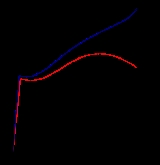xTensile strengthOverview

Ultimate tensile strength (UTS), often shortened to tensile strength (TS) or ultimate strength, is the maximum stress that a material can withstand while being stretched or pulled before necking
Necking (engineering)
Necking, in engineering or materials science, is a mode of tensile deformation where relatively large amounts of strain localize disproportionately in a small region of the material. The resulting prominent decrease in local cross-sectional area provides the basis for the name "neck"...

, which is when the specimen's cross-section
Cross section (geometry)
In geometry, a cross-section is the intersection of a figure in 2-dimensional space with a line, or of a body in 3-dimensional space with a plane, etc...

starts to significantly contract. Tensile strength is the opposite of compressive strength
Compressive strength
Compressive strength is the capacity of a material or structure to withstand axially directed pushing forces. When the limit of compressive strength is reached, materials are crushed. Concrete can be made to have high compressive strength, e.g...

and the values can be quite different.

The UTS is usually found by performing a tensile test and recording the stress versus strain; the highest point of the stress-strain curve
Stress-strain curve
During tensile testing of a material sample, the stress–strain curve is a graphical representation of the relationship between stress, derived from measuring the load applied on the sample, and strain, derived from measuring the deformation of the sample, i.e. elongation, compression, or distortion...

is the UTS.When designing a steel structure the top of beam would be in tension and the bottom in compression. what is more critical doing stress calcs.?For my idea I need to have a high uts of 0.63"(d)solid plastic what would be the best plastic in psi's to use?Why is tensile strength test conducted?What stands for RmWhat is the ultimate tensile strength of pure aluminium?DiscussionsTensil Strength